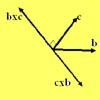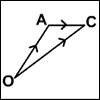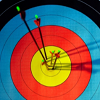Search by Topic

Resources tagged with Vectors similar to Cross with the Scalar Product:

Filter by: Content type:
Age range:
Challenge level:

There are 24 results

Broad Topics > Vectors and Matrices > VectorsMatrix Meaning

Age 16 to 18 Challenge Level:

Explore the meaning behind the algebra and geometry of matrices with these 10 individual problems.Polygon Walk

Age 16 to 18 Challenge Level:

Go on a vector walk and determine which points on the walk are closest to the origin.Nine Eigen

Age 16 to 18 Challenge Level:

Explore how matrices can fix vectors and vector directions.Vector Walk

Age 14 to 18 Challenge Level:

Starting with two basic vector steps, which destinations can you reach on a vector walk?Fix Me or Crush Me

Age 16 to 18 Challenge Level:

Can you make matrices which will fix one lucky vector and crush another to zero?Air Routes

Age 16 to 18 Challenge Level:

Find the distance of the shortest air route at an altitude of 6000 metres between London and Cape Town given the latitudes and longitudes. A simple application of scalar products of vectors.Multiplication of Vectors

Age 16 to 18

An account of multiplication of vectors, both scalar products and vector products.From Point to Point

Age 14 to 16 Short Challenge Level:

Can you combine vectors to get from one point to another?Coordinated Crystals

Age 16 to 18 Challenge Level:

Explore the lattice and vector structure of this crystal.An Introduction to Vectors

Age 14 to 18

The article provides a summary of the elementary ideas about vectors usually met in school mathematics, describes what vectors are and how to add, subtract and multiply them by scalars and indicates. . . .V-P Cycles

Age 16 to 18 Challenge Level:

Form a sequence of vectors by multiplying each vector (using vector products) by a constant vector to get the next one in the seuence(like a GP). What happens?Age 16 to 18 Challenge Level:

A quadrilateral changes shape with the edge lengths constant. Show the scalar product of the diagonals is constant. If the diagonals are perpendicular in one position are they always perpendicular?8 Methods for Three by One

Age 14 to 18 Challenge Level:

This problem in geometry has been solved in no less than EIGHT ways by a pair of students. How would you solve it? How many of their solutions can you follow? How are they the same or different?. . . .The Use of Mathematics in Computer Games

Age 16 to 18

An account of how mathematics is used in computer games including geometry, vectors, transformations, 3D graphics, graph theory and simulations.Which Twin Is Older?

Age 16 to 18

A simplified account of special relativity and the twins paradox.Vector Journeys

Age 14 to 18 Challenge Level:

Charlie likes to go for walks around a square park, while Alison likes to cut across diagonally. Can you find relationships between the vectors they walk along?Spotting the Loophole

Age 14 to 16 Challenge Level:

A visualisation problem in which you search for vectors which sum to zero from a jumble of arrows. Will your eyes be quicker than algebra?Vector Racer

Age 11 to 16 Challenge Level:

The classic vector racing game.Another Triangle in a Triangle

Age 16 to 18 Challenge Level:

Can you work out the fraction of the original triangle that is covered by the green triangle?Vector Gem Collector

Age 14 to 18 Challenge Level:

Use vectors to collect as many gems as you can and bring them safely home!Areas of Parallelograms

Age 14 to 16 Challenge Level:

Can you find the area of a parallelogram defined by two vectors?Cubestick

Age 16 to 18 Challenge Level:

Stick some cubes together to make a cuboid. Find two of the angles by as many different methods as you can devise.